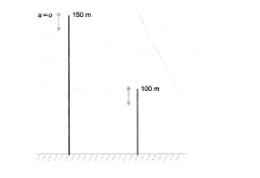# An object is dropped from rest at the height of`
Question:

An object is dropped from rest at the height of $150 \mathrm{~m}$ and simultaneously another object is dropped from

rest at the height of $100 \mathrm{~m}$.

What is the difference in their heights after $2 \mathrm{~s}$ if both the objects drop with same accelerations? How does

the difference in heights vary with time?

Solution:

Distance moved by the object dropped from height of $150 \mathrm{~m}$ in 2 seconds can be calculated using

$\mathrm{S}=u t+\frac{1}{2} a t^{2}$

Here, $u=0, a=g=10 \mathrm{~ms}^{-2}$

$\therefore \quad S=\frac{1}{2} \times 10 \times 4=20 \mathrm{~m}$Height of the object from ground after 2 seconds $=150-20=130 \mathrm{~m}$ Similarly, distance moved by the other

object in 2 seconds $=20 \mathrm{~m}$

Height of the second object from ground after 2 seconds $=100-20=80 \mathrm{~m}$

$\therefore$ Difference in their heights after 2 seconds $=130 \mathrm{~m}-80 \mathrm{~m}=50 \mathrm{~m}$

Difference in height of both objects remains the same with time.